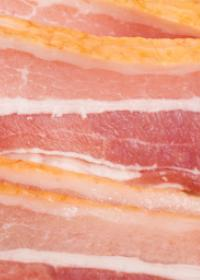This vignette tests pkgdown output rendering for several use cases.

Yay1

## Figures

plot(1:10)## External files

x <- readLines("test.txt")
x
#>  "a" "b" "c" "d"## Details tag

This should only be shown when required
Multiple paragraphs

First paragraph

Second paragraph
Some R code
1 + 2
#>  3

## Tables

col 1 col 2 col 3 col 4
Brightness Total brightness, total reflectance, spectral intensity $y = x^2$ test

## Mathjax

$f(x) = \dfrac{1}{\sqrt{2\pi\sigma^2}} e^{-\frac{(x-\mu^2)}{2\sigma^2}}$

Inline equations: $$y=x^2$$

## Code

### Line width

pkgdown:::ruler()
#> ----+----1----+----2----+----3----+----4----+----5----+----6----+----7----+----8
#> 12345678901234567890123456789012345678901234567890123456789012345678901234567890

cat(rep("x ", 100), sep = "")
#> x x x x x x x x x x x x x x x x x x x x x x x x x x x x x x x x x x x x x x x x x x x x x x x x x x x x x x x x x x x x x x x x x x x x x x x x x x x x x x x x x x x x x x x x x x x x x x x x x x x x
cat(rep("xy", 100), sep = "")
#> xyxyxyxyxyxyxyxyxyxyxyxyxyxyxyxyxyxyxyxyxyxyxyxyxyxyxyxyxyxyxyxyxyxyxyxyxyxyxyxyxyxyxyxyxyxyxyxyxyxyxyxyxyxyxyxyxyxyxyxyxyxyxyxyxyxyxyxyxyxyxyxyxyxyxyxyxyxyxyxyxyxyxyxyxyxyxyxyxyxyxyxyxyxyxyxyxyxyxyxy

### Should be highlighted

Valid R code in \preformatted{}:

mean(a + 1)

R code in R block:

mean(a + 1)

R code in r block:

mean(a + 1)

Yaml

yaml: [a, 1]

### Shouldn’t be highlighted

Non-R code in \preformatted{}

yaml: [a, b, c]

### Crayon

cat(cli::col_red("This is red"), "\n")
#> This is red
cat(cli::col_blue("This is blue\n"), "\n")
#> This is blue
#>

message(cli::col_green("This is green"))
#> This is green

warning(cli::style_bold("This is bold"))
#> Warning: This is bold

Some text

stop(cli::style_italic("This is italic"))
#> Error in eval(expr, envir, enclos): This is italic

Some more text

## Quoted text

Flush
1 space indent
2 space indent
3 space indent

## This section is unnumbered

There should however be no bug here!

## Tabsets

### Tabset with pills

blablablabla

1 + 1

Stuff

blop

### Tabset without pils

something nice

plot(1:42)This tab should be active

Hello!

Bonjour!

Guten tag.